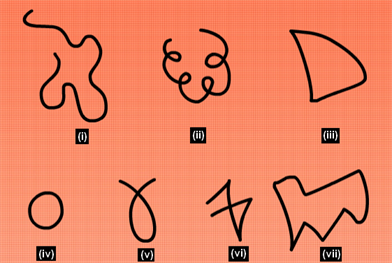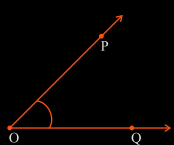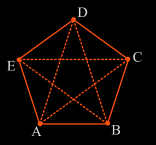# Polygon, Curve And Angle

Geometry is the most practical branch of mathematics that deals with shapes and sizes of figures and their properties. Geometry can be categorized into Plane and Solid Geometry. Plane geometry deals with flat shapes like lines, curves, polygons, etc., that can be drawn on a piece of paper. On the other hand, solid geometry is about three-dimensional objects such as cylinders, cubes, spheres, etc. Here we shall discuss a few fundamental elements of plane geometry – curve, angle and polygon.

## Curve

A curve is a smoothly flowing line that you can draw on a paper without using a ruler. The random lines that you doodle on paper are also curves.

There are three types of the curve:

• Simple curves: Curve that does not intersect itself at any point. In the figure, (i), (iii), (iv) and (vii) are simple curves.
• Open curves: Curve whose end points do not meet. (i), (ii), (v) and (vi) are open curves.
• Closed curves: Curve that completely encloses an area and has no endpoints. (iii), (iv) and (vii) are open curves.Types of Curves

## Angle

An angle is an inclination of two rays that emerges from a common point. This point is called the vertex of the angle and the two rays forming the angle are called its arms or sides.

Note:

• If the arms form an angle of 90 degrees between them, it is called a right angle.
• If the inclination between the arms is less than a right angle, it is called an acute angle.
• If the inclination between the arms is more than a right angle, it is called an obtuse angleAn Angle

In the following figure:

• O is the vertex of the angle.
• OP and OQ are the rays forming the angle. They are the sides or arms of the angle.
• When naming an angle, the vertex is always written as the middle letter. Here the angle is written as ∠POQObtuse, Right and Acute Angle

## Polygon

A polygon is a flat figure enclosed by three or more line segments. The line segments are called the sides of the polygon. The point where two line segments meet is called the vertex of the polygon. The pair of sides that meet at the same vertex are called adjacent sides. The endpoints of the same side are called the adjacent vertices. The line segment joining two non-adjacent vertices of a polygon is called a diagonal of the polygon.

• When all the sides are equal in length, the polygon is said to be a regular polygon. E.g., square. Otherwise, it is an irregular polygon.
• A triangle is a polygon with a minimum number of sides, which is 3.
• Their nomenclature is done on the basis of the number of sides they have. A polygon with 3 sides is called a triangle, with 4 sides is called a quadrilateral and with 5 sides is called a pentagon, and so on.A Polygon

In the following polygon:

• AB, BC, CD, DE and EA are the sides
• A, B, C, D and E are the vertices
• AB and BC are the adjacent sides
• A and E are the adjacent vertices. A and C are not the adjacent vertices
• AD, AC, BE, BD and CE are the diagonals